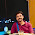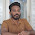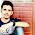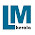Menu

# How to Find the Percentage of Mark?You know how to find the percentage of marks obtained from examination?. If you don't know, i will explain with simple calculator. There are different medium to calculate % of marks., example: calculator, digital medias etc. Calculation of percentage of mark using common formula,

X/Y x 100/1 = "% Mark" or X/Y x 100 = "% Mark"

There "X" is your total mark and Y is maximum mark.

If it difficulty to calculate, then see our instant percentage calculator. This calculator can also use for your maths study material.

 Percentage Calculator Your Total Marks Max. Mark Answer: %

Post your doubt and feedback's in the below comment section.

#### Post a Comment

1.thanks for easlly Calc my percentage.....!

1.2.Awesome Bro......

1.3.Thanks Bro...

4.How to check maximum mark

5.How to check maximum mark

1.Maximum mark is 'out of mark'.

6.WHAT IS THE TOTAL MARK IN HSE

7.nizee calculation method,thnks

8.9.My total mark is 80 what is the percentage in sslc

1.Your maximum marks?
80 mark is out of how much mark?

10.Here are the types of charts. There is a hip to waist calculator. That's a ratio. If the waist divided by the hips is greater than .8, it indicates a health risk. There's a body frame calculator, which you'll need help. You measure wrist and elbow joint. There's some formula and you can tell your frame. You can get BMI. For individual measurements against an ideal, you look up dressmaking sites, and there you'll see the ideal for every single aspect for fitting clothing. You'll be able to tell where you deviate from what is ideal for pattern makers. For example, if ideal arm length is 22, and yours is 22.5", that is .5" difference which would need to be added to the pattern. Now, what you seem to want is to know your body fat ratio. This is not online, but done in a variety of ways in a setting. I suggest you Percentage calculator through this you get more results about How to calculate percentage .

Top